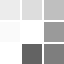Rainbow Numbers

• €34.95
• Ex Tax: €28.41
Set of 155 magnetic foam mathematics pieces with unique in-built magnetic board. Rainbow Numbers combines simple mathematical concepts so children can keep track of counting, see number relationships and understand place value. Concrete objects are used to model what is happening when performing a m..

Set of 155 magnetic foam mathematics pieces with unique in-built magnetic board. Rainbow Numbers combines simple mathematical concepts so children can keep track of counting, see number relationships and understand place value. Concrete objects are used to model what is happening when performing a mathematical operation such as addition or subtraction. Perfect for meeting Common Core Standards - Number & Operations in Base Ten. Includes: 60 x counting dots, 11 x frames, 40 x numbers, 6 x equations, 21 x base tens, 2 x printed frames, 2 x dividers, 3 x place values. Activity guide. Ages 4+

Write a review

Note: HTML is not translated!